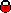## Measurement and NLO DGLAP QCD Interpretation of Diffractive Deep-Inelastic Scattering at HERA

 Reference F-P Schilling, talk presented at DIS 2002, April 2002 Document Conference paper Figures (1)  (2)  (3)  (4)  (5)  (6)  (7a)  (7b)  (8a)  (8b)  (9)  (10)  (11)  (12)  (13)  (14)  (15)  (16)  (17)  (18a)  (18b)  (19a)  (19b)  (20)  (A1a)  (A1b)  (A2a)  (A2b)  (A3a)  (A3b)  (A4)  (A5)  (A6)  (A7)  (A8)  (A9)  (A10)  (A11)  (A12)  (A13)  (A14) Tables Contact P Newman, F Schilling Abstract A high precision inclusive measurement of the diffractive deep inelastic scattering (DIS) process ep ightarrow eXY is presented, where Y is a proton or a low mass proton excitation carrying a fraction 1 - xpom > 0.95 of the beam longitudinal momentum and the squared 4-momentum transfer at the proton vertex t > -1 GeV^2. The measurement, based on an integrated luminosity of 10.6 pb-1, is presented in the form of a diffractive reduced cross section sigma_r^{D(3)}, measured in the kinematic range 6.5 \leq Q^2 \leq 120 GeV^2, 0.01 \leq eta \leq 0.9 and 10^{-4} < xpom < 0.05. The ratio of the diffractive to the inclusive cross section sigma_r^{D(3)} (x, Q^2, xpom) / sigma_r (x, Q^2) is measured and found to be remarkably flat as a function of Q^2 with x and xpom fixed. The measured cross section is compared with various models for diffractive DIS. The xpom dependence of the data is interpreted in terms of a measurement of the effective pomeron intercept alphapom(0) = 1.173 \pm 0.018 (stat.) \pm 0.017 (syst.) +0.063/-0.035 (model). A NLO DGLAP QCD fit is performed to the data, together with an assessment of the experimental and theoretical uncertainties on the resulting diffractive parton densities. The diffractive exchange is shown to be dominated by the diffractive gluon density, which carries an integrated fraction 75 \pm 15% of the exchanged momentum at Q^2=10 GeV^2 and extends to large fractional momenta. The parton densities are used to make updated comparisons with diffractive dijet and open charm cross sections at HERA and the Tevatron, thus testing the factorisation properties of hard diffraction. Comments . Links back to overview listingH1 internal information